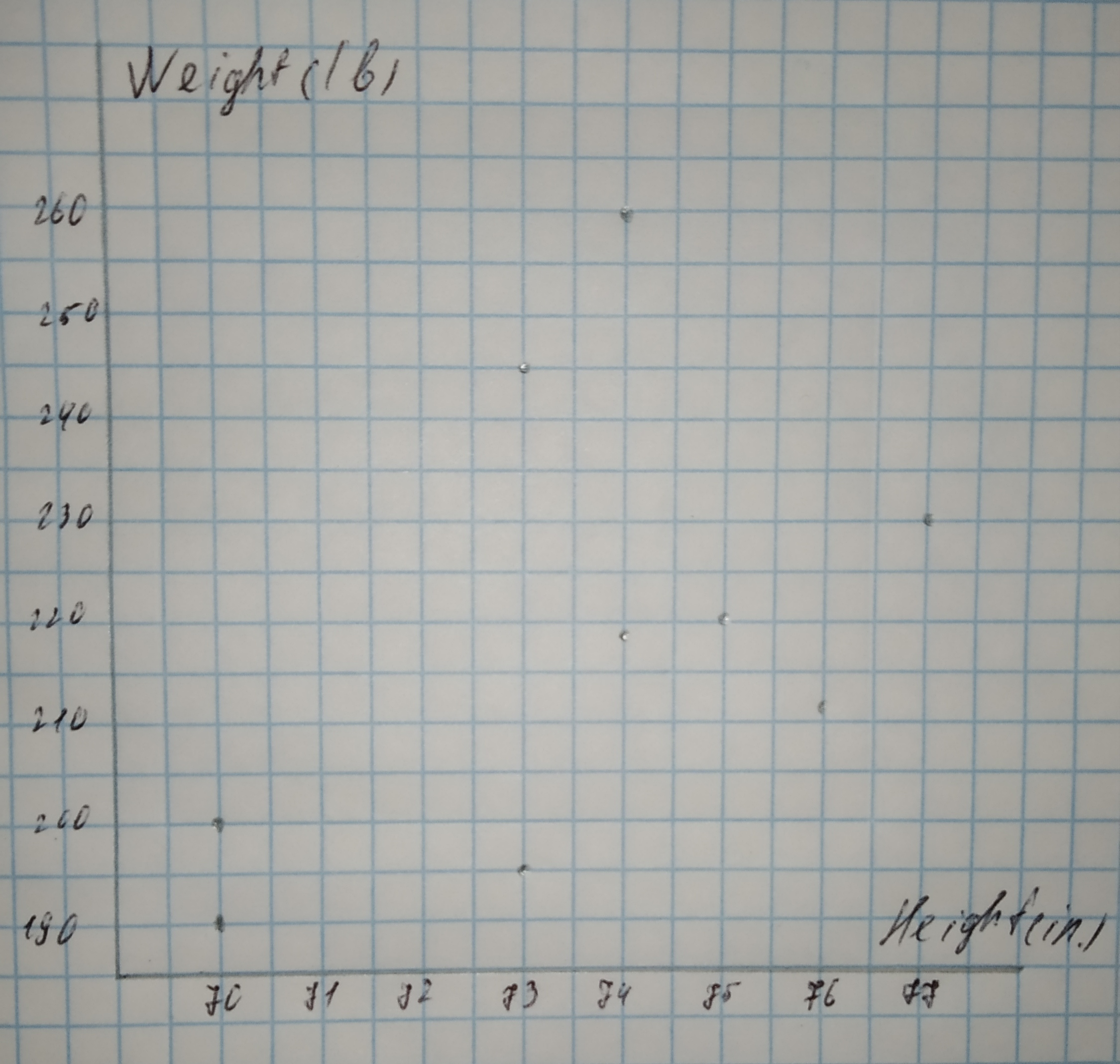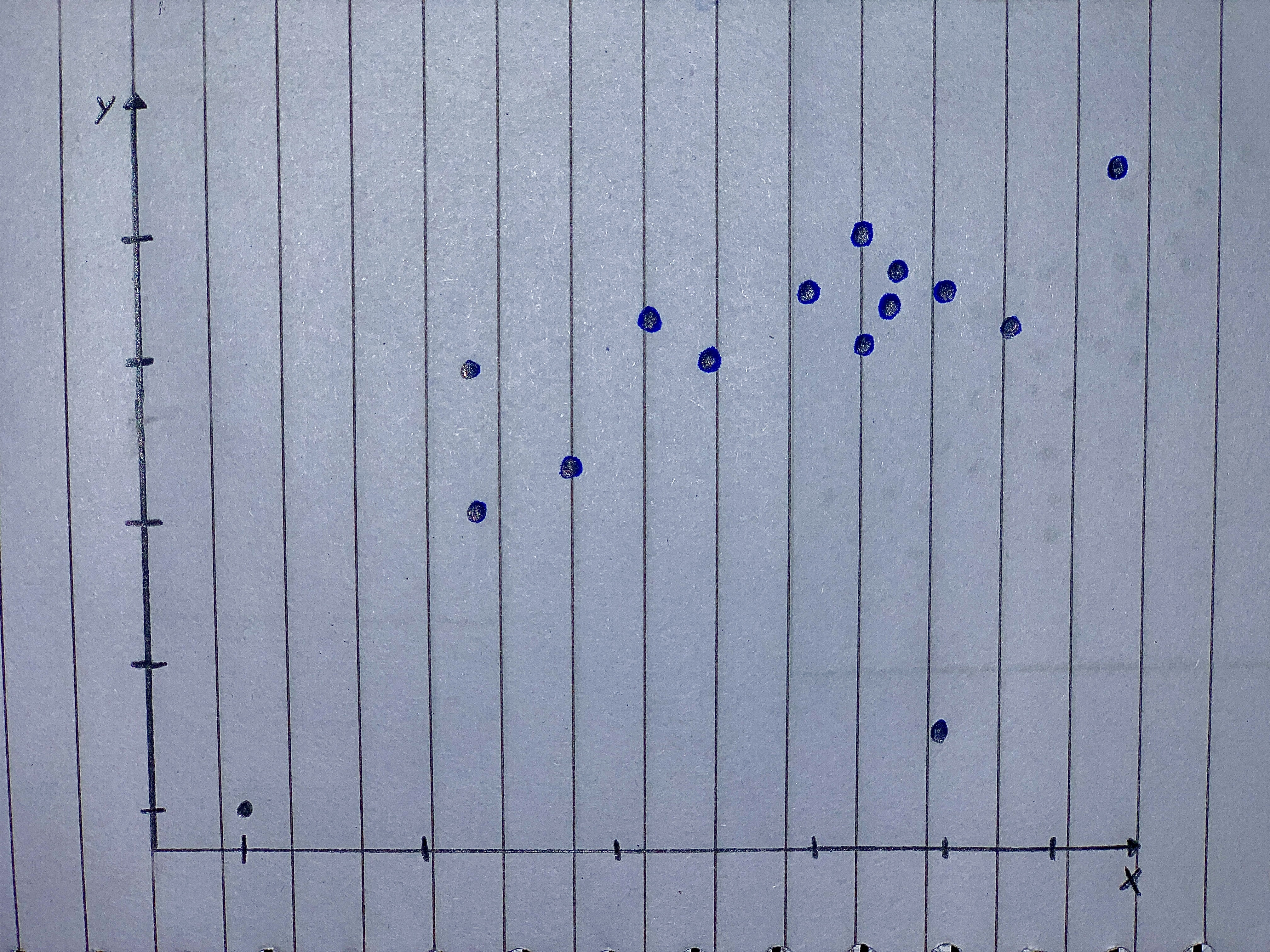Ask question

# a. Make a scatterplot for the data in the table below.Height and Weight of Football Players# a. Make a scatterplot for the data in the table below.Height and Weight of Football Players

Question
Scatterplotsasked 2021-02-25

a. Make a scatterplot for the data in the table below.
Height and Weight of Football Players
$$\begin{array}{|c|c|}\hline \text{Height (in.):} & 77 & 75 & 76 & 70 & 70 & 73 & 74 & 74 & 73 \\ \hline \text{Weight (lb):} & 230 & 220 & 212 & 190 & 201 & 245 & 218 & 260 & 196 \\ \hline \end{array}$$

b. Which display - the table or the scatter plot - do you think is a more appropriate display of the data? Explain your reasoning.

## Answers (1)2021-02-26
Step 1
(a) Scatterplot
Height is on the horizontal axis and Weight is on the vertical axis.
The heights range from 70 to 77, thus an appropriate scale for the horizontal axis is from 69 to 78
The weights range from 190 to 260, thus an appropriate scale for the vertical axis is from 180 to 270.Step 2
(b) The scatterplot is a more appropriate display of the data, because the relationship between height and weight is more obvious from the scatterplot compared to the table.
For example, we note that the weight tends to increase as the height increases, because the pattern in the scatterplot slopes upwards. However, this fact would have been much harder to derive from the table.
Result:
(a) Height is on the horizontal axis and Weight is on the vertical axis.
(b) Scatter plot.

### Relevant Questionsasked 2021-03-06

Make a scatterplot for the data.
Height and Weight of Females
$$\begin{array}{|c|c|}\hline \text{Height (in.):} & 58 & 60 & 62 & 64 & 65 & 66 & 68 & 70 & 72 \\ \hline \text{Weight (lb):} & 115 & 120 & 125 & 133 & 136 & 115 & 146 & 153 & 159 \\ \hline \end{array}$$asked 2021-05-31
Make a scatterplot for the data.
Height and Weight of Females
Height (in.): 58, 60, 62, 64, 65, 66, 68, 70, 72
Weight (lb): 115, 120, 125, 133, 136, 115, 146, 153, 159asked 2021-02-19

Make a scatterplot for each set of data. Tell whether the data show a linear association or a nonlinear association.
$$(1,\ 2),\ (7,\ 9.5),\ (4,\ 7),\ (2,\ 4.2),\ (6,\ 8.25),\ (3,\ 5.8),\ (5,\ 8),\ (8,\ 10),\ (0,\ 0)$$asked 2021-01-31

Make a scatterplot for each set of data.
$$\begin{array}{|c|c|}\hline \text{Hits:} & 7 & 8 & 4 & 11 & 8 & 2 & 5 & 9 & 1 & 4 \\ \hline \text{Runs:} & 3 & 2 & 2 & 7 & 4 & 2 & 1 & 3 & 0 & 1 \\ \hline \end{array}$$asked 2021-01-13

For each set of data below, draw a scatterplot and decide whether or not the data exhibits approximately periodic behaviour.

a) $$\begin{array}{|c|c|}\hline x & 0 & 1 & 2 & 3 & 4 & 5 & 6 & 7 & 8 & 9 & 10 & 11 & 12 \\ \hline y & 0 & 1 & 1.4 & 1 & 0 & -1 & -1.4 & -1 & 0 & 1 & 1.4 & 1 & 0 \\ \hline \end{array}$$

b) $$\begin{array}{|c|c|}\hline x & 0 & 1 & 2 & 3 & 4 \\ \hline y & 4 & 1 & 0 & 1 & 4 \\ \hline \end{array}$$

c) $$\begin{array}{|c|c|}\hline x & 0 & 0.5 & 1.0 & 1.5 & 2.0 & 2.5 & 3.0 & 3.5 \\ \hline y & 0 & 1.9 & 3.5 & 4.5 & 4.7 & 4.3 & 3.4 & 2.4 \\ \hline \end{array}$$

d) $$\begin{array}{|c|c|}\hline x & 0 & 2 & 3 & 4 & 5 & 6 & 7 & 8 & 9 & 10 & 12 \\ \hline y & 0 & 4.7 & 3.4 & 1.7 & 2.1 & 5.2 & 8.9 & 10.9 & 10.2 & 8.4 & 10.4 \\ \hline \end{array}$$asked 2021-01-08

Make a scatterplot of the data and graph the function $$\displaystyle{f{{\left({x}\right)}}}=\ -{8}{x}^{{{2}}}\ +\ {95}{x}\ +\ {745}.$$ Make a residual plot and describe how well the function fits the data. $$\begin{array}{|c|c|} \hline \text{Price Increase} & 0 & 1 & 2 & 3 & 4 \\ \hline \text{Sales} & 730 & 850 & 930 & 951 & 1010 \\ \hline \end{array}$$asked 2021-02-03

The following data on = soil depth (in centimeters) and y = percentage of montmorillonite in the soil were taken from a scatterplot in the paper "Ancient Maya Drained Field Agriculture: Its Possible Application Today in the New River Floodplain, Belize, C.A." (Agricultural Ecosystems and Environment : 67-84):
a. Draw a scatterplot of y versus x.
b. The equation of the least-squares line is 0.45x. Draw this line on your scatterplot. Do there appear to be any large residuals?
c. Compute the residuals, and construct a residual plot. Are there any unusual features in the plot?
$$\begin{array}{|c|c|}\hline x & 40 & 50 & 60 & 70 & 80 & 90 & 100 \\ \hline y & 58 & 34 & 32 & 30 & 28 & 27 & 22 \\ \hline \end{array}$$
$$\displaystyle{\left[\hat{{{y}}}={64.50}\right]}$$.asked 2020-12-05

Use the sample data to construct a scatterplot.
Use the first variable for the x-axis. Based on the scatterplot, what do you conclude about a linear correlation?
The table li sts che t sizes (di stance around chest in inches) and weights (pounds) of anesthetized bears that were measured.
$$\begin{array}{|c|c|}\hline \text{Chest(in.)} & 26 & 45 & 54 & 49 & 35 & 41 & 41 \\ \hline \text{Weight(lb)} & 80 & 344 & 416 & 348 & 166 & 220 & 262 \\ \hline \end{array}$$asked 2020-11-29

Straight Lines Graph the straight lines in Exercises 1–3.
Then find the change in y for a one-unit change in x, find the point at which the line crosses the y-axis, and calculate the value of y when x 52.5.
1. $$y x\ 5\ 1\ 2.0\ 0.5$$
2. $$y x\ 5\ 1\ 40\ 36.2$$
3. $$y x\ 5\ 25\ 6$$
Scatterplots For the scatterplots in Exercises 5 describe the pattern that you see. How strong is the pattern? Do you see any outliers or clusters?asked 2021-03-06
From the Statistical Abstract of the United States, we obtained data on percentage of gross domestic product (GDP) spent on health care and life expectancy, in years, for selected countries. a) Obtain a scatterplot for the data. b) Decide whether finding a regression line for the data is reasonable. If so, then also do parts (c)-(f). c) Determine and interpret the regression equation for the data. d) Identify potential outliers and influential observations. e) In case a potential outlier is present, remove it and discuss the effect. f) In case a potential influential observation is present, remove it and discuss the effect.
...